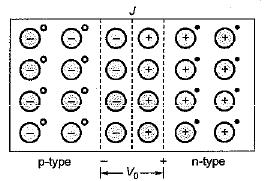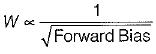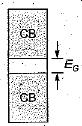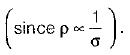Courses

# Semiconductor Diodes

## 10 Questions MCQ Test Topicwise Question Bank for GATE Electrical Engineering | Semiconductor Diodes

Description
This mock test of Semiconductor Diodes for Electrical Engineering (EE) helps you for every Electrical Engineering (EE) entrance exam. This contains 10 Multiple Choice Questions for Electrical Engineering (EE) Semiconductor Diodes (mcq) to study with solutions a complete question bank. The solved questions answers in this Semiconductor Diodes quiz give you a good mix of easy questions and tough questions. Electrical Engineering (EE) students definitely take this Semiconductor Diodes exercise for a better result in the exam. You can find other Semiconductor Diodes extra questions, long questions & short questions for Electrical Engineering (EE) on EduRev as well by searching above.
QUESTION: 1

### The barrier voltage (V0 or Vr) in a junction diode is the effect of

Solution:Due to the density gradient across the junction, holes will initially diffuse to the right across the junction, and electrons to the left. The positive holes which neutralizes the acceptor ions near the junction in the p-type silicon disappears as a result of combination with electrons which have diffused across the junction. Similarly, electrons in n-type semiconductor combines with holes.

QUESTION: 2

### In a zener diode large reverse current is due to

Solution:

The large reverse current flow in zener diode is due to rupturing-off or tearing-off of covalent bends within the depletion layer. This phenomena is called zener breakdown phenomena, It is due to large electric field intensity.

QUESTION: 3

### For thermionic emission

Solution:

The work function of a metal represents the minimum amount of energy that must be given to the fastest-moving electron at the absolute zero of temperature in order for this electron to be able to escape from the metal. Hence, low work function (Ew) is required for thermionic emission.

QUESTION: 4

The most commonly used type of electron emission in electron tubes is

Solution:
QUESTION: 5

When forward bias is applied to a junction diode, it

Solution:

Width of depletion layer,Hence, when forward bias is applied to a p-n junction diode, width of the depletion layer is reduced i.e. potential barrier is decreased

QUESTION: 6

The emission of electrons in a vacuum diode is achieved by

Solution:
QUESTION: 7

The main reason why electrons can tunnel through a p-n junction is that

Solution:

In a p-n junction tunnel diode, the depletion layer width is negligible and therefore, the charge carriers will be penetrating the depletion layer almost at the speed of light and this property is called tunnelling of charge carriers across the junction.

QUESTION: 8

The forbidden energy gap in semiconductors

Solution:

The energy level diagram for a semiconductor is shown below.EG is called “band gap” or "forbidden energy gap” which lies between the valence band and the conduction band.

QUESTION: 9

The barrier potential for a Ge p-n junction is

Solution:

Typical value of “Barrier voltage” or "Contact potential” for a p-n junction is:
V0 = 0.2 V (for Ge p-n junction diode)
= 0.7V (for Si p-n junction diode)

QUESTION: 10

With the increase in temperature, the resistivity of an intrinsic semiconductor decreases. This is because, with the increase of temperature

Solution:

In an intrinsic semiconductor, as the temperature increases mobility is slightly reduced since mobility of charge carrier is inversely proportional to temperature. This will slightly reduce the conductivity. But, due to temperature effect, large number of covalent bonds are broken which creates electrons and holes and this increases the conductivity by a larger value. As a result of this resistivity is decreased